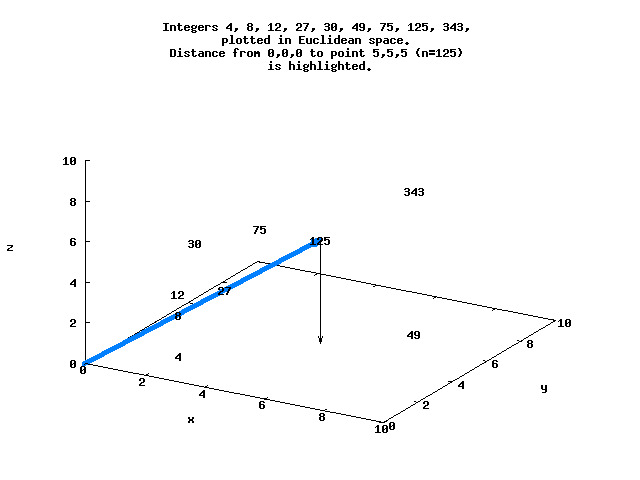## Integers in Euclidean space order

Consider the factored integers

`N = [(0), (1), (2), (3), (2,2), 5, (2,3), 7, (2,2,2)...]`

with each factor representing a point in Euclidean space. Each of these points is at a distance d from the origin, and can be ordered by d.

```-- Ieso.hs
-- Integers in Euclidean space order

-- Uses the Haskell Number Theory functions, from the "Haskell for Maths"
import Data.List
import Primes

-- Return distance between two points in Euclidean space. Points are lists
-- of possibly unequal lengths, padded with zeros as needed.
dist_points x y = sqrt (realToFrac ((foldl (+) 0 (map sq (zipWith (-) x1 y1)))))
where x1 = pad (lengthLongest [x,y]) x
y1 = pad (lengthLongest [x,y]) y
sq x = x * x
lengthLongest l = maximum (map length l)
where zeroPadding = genericTake (len - genericLength x) (repeat 0)

-- Return list of all prime factors of n
primeFactors 1 = 
primeFactors n = flatten (map expandedFactors (primePowerFactors n))
where
expandedFactors x = genericTake (snd x) (repeat (fst x))
flatten :: [[a]] -> [a]
flatten = foldl (++) []

-- Return distance between two factored integers
distance n m = dist_points (primeFactors n) (primeFactors m)

-- Return list of distances between origin and integers up to n,
-- sorted by distance d. Where ties occur, the lowest n comes first, i.e.,
-- where d=5 for both n=48 and n=5, the order is ... 5, 48 ....
-- Neil Sloane has suggested a different way of breaking ties is use of
-- lexicographic order, ie for n=48 [2,2,2,3] comes before n=5  - see
-- below.
distanceOrigin n = map snd (sort d)
where
d = [(distance 0 i, i) | i <- [0..n]]

-- In order to list the integers within a certain distance d from the origin,
-- we have to calculate distances up to 2^(d^2/4), e.g. to list ALL
-- integers within a distance of 10, use range 2...2^25.

-- I believe a list is "complete" up to where a power of 2 appears,
-- because numbers greater than that power of 2 can't be closer
-- to the origin (except ties, depending on they are handled). However,
-- I am curious about how to determine the  minimum number of
-- integers you have to calculate in order to make a list complete,
-- e.g., how high do we have to look to be sure we've found the
-- 20 integers closest to the origin?

main = do
-- Calculate integers within ~7 units of origin
print "n ordering for ties"
let d = [(distance 0 i, i) | i <- [0..8192]]
print (map snd (sort d))

-- Recalculate using lexicographic order to break ties
print "lexicographical ordering for ties"
let l = [(distance 0 i, primeFactors i, i) | i <- [0..8192]]
print (map lastx (sort l))
where
lastx (x, y, z) = z
```

For example, d=8.66 for N=125 at the point (5,5,5), while d=5.29 for N=128 at (2,2,2,2,2,2,2) :

```*Ieso> distance 0 125
8.660254037844387
*Ieso> distance 0 128
5.291502622129181
```

Here is a simple view of several of these integers that lie in 3-space, with the distance between the origin and N = 125 highlighted.The sequence N=[0..8192] in Euclidean space ordered by d begins

```*Ieso> distanceOrigin 8192
0,1,2,4,3,8,6,16,12,9,32,24,18,64,5,48,36,27,128,10,96,72,54,256,20,192,15,144,108,81,512,40,384,30,288,216,162,1024
```

(One way to think about this may be that integers with higher Ω(n) should be relatively clustered around the origin).

(The sequence is listed as OEIS A168521).

Posted in mathematics | Comments Off

## Units for Android 1.0 released

This is a port of standard GNU units to the Android platform.

Posted in android | Comments Off

## PDF MD Encrypt for Android

This app creates and emails an (optionally encrypted) PDF file from your Android phone/device.

Potentially useful for physicians and other professionals, this allows you to communicate in a secure manner with patients. For example, you can easily jot down instructions (e.g., for taking medication) on your Android device and provide those instructions to your patient securely by email.

Posted in android, medicine | Comments Off